# R S Aggarwal Solutions for Class 11 Maths Chapter 27 Limits Exercise 27B

Exercise 27B of Chapter 27 – Limits, mainly deals with the trigonometric limits. This set of solutions will help students in understanding the important steps involved in finding the limits of various trigonometric functions. Students can download R S Aggarwal Solutions for Class 11 chapter 27 exercise 27B solutions from the link given below.

## Download PDF of R S Aggarwal Solutions for Class 11 Maths Chapter 27 Limits Exercise 27B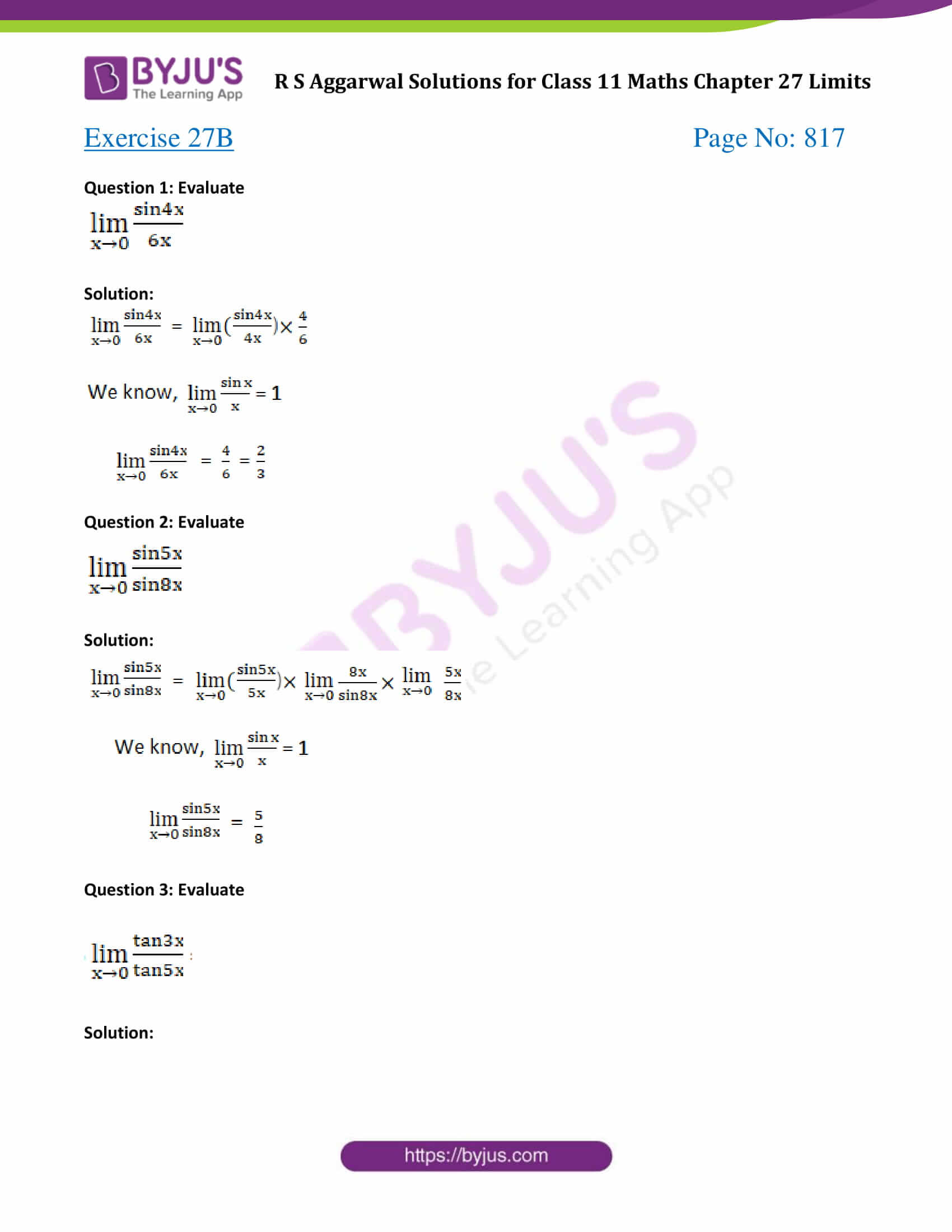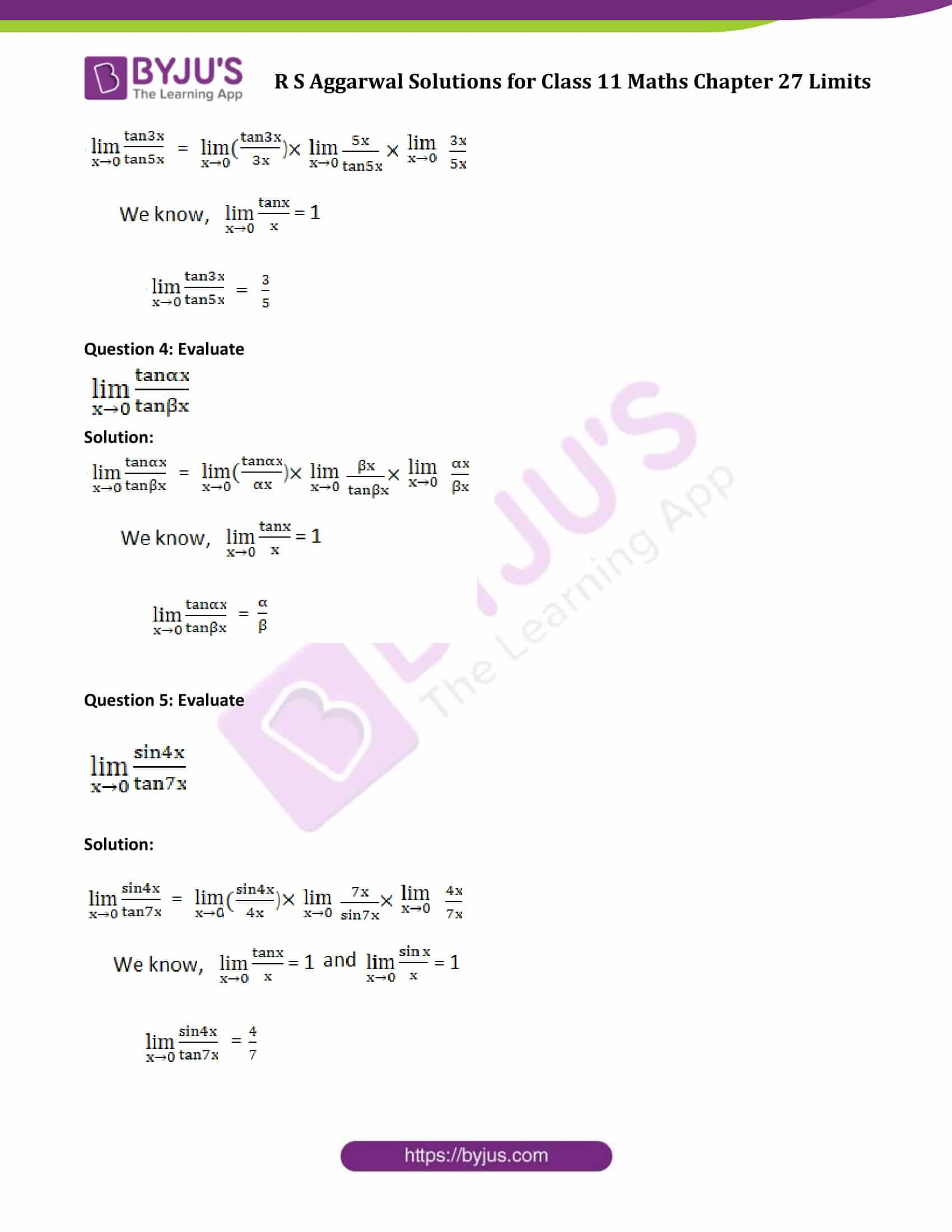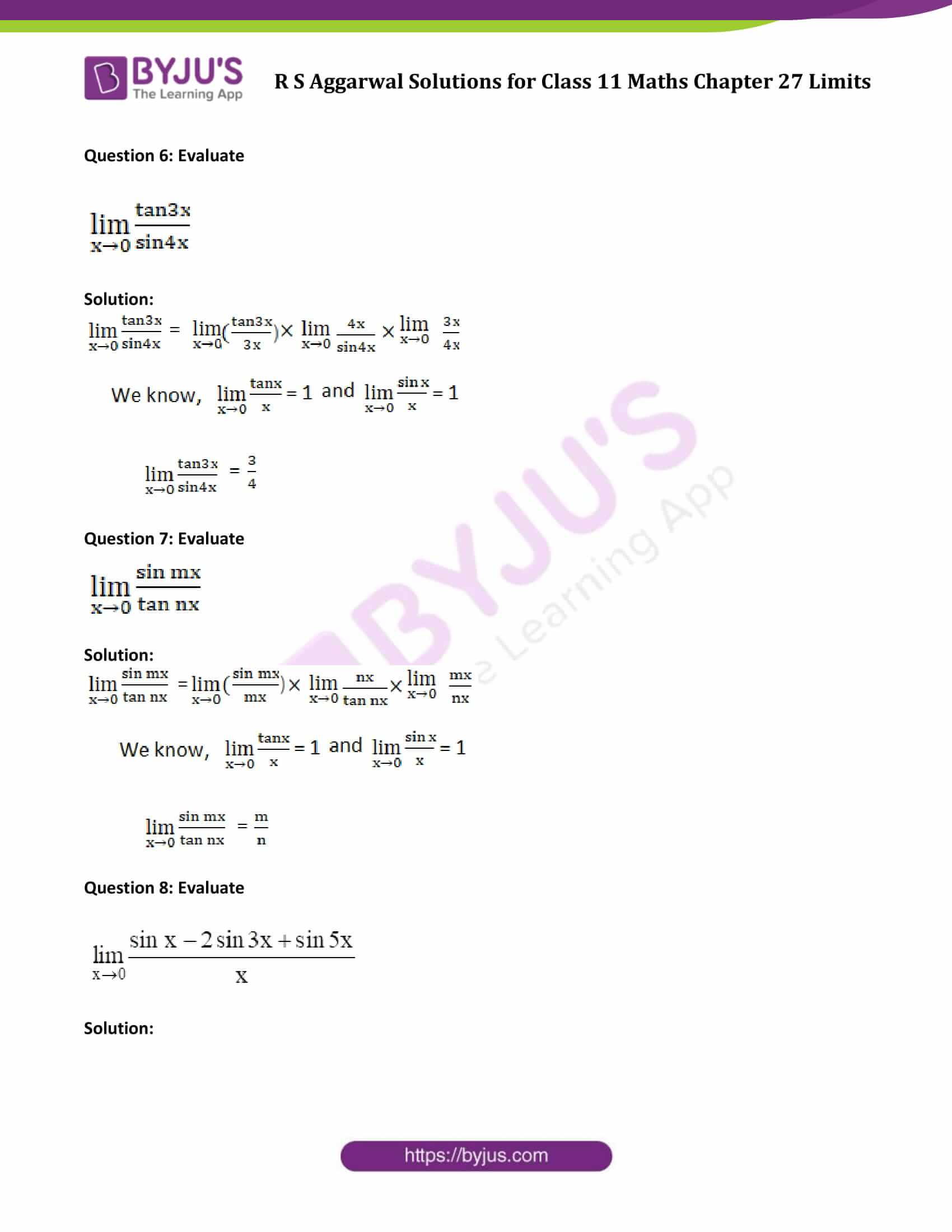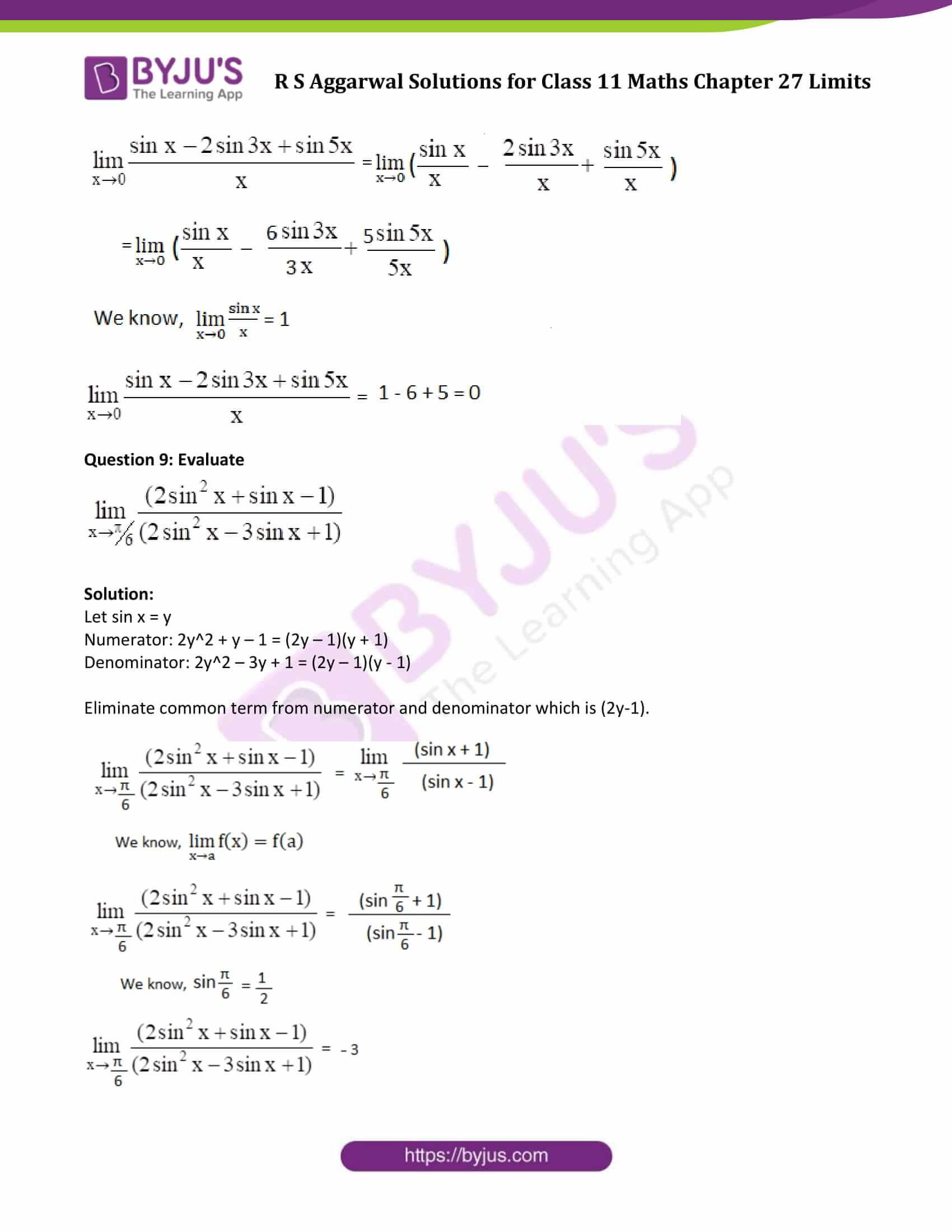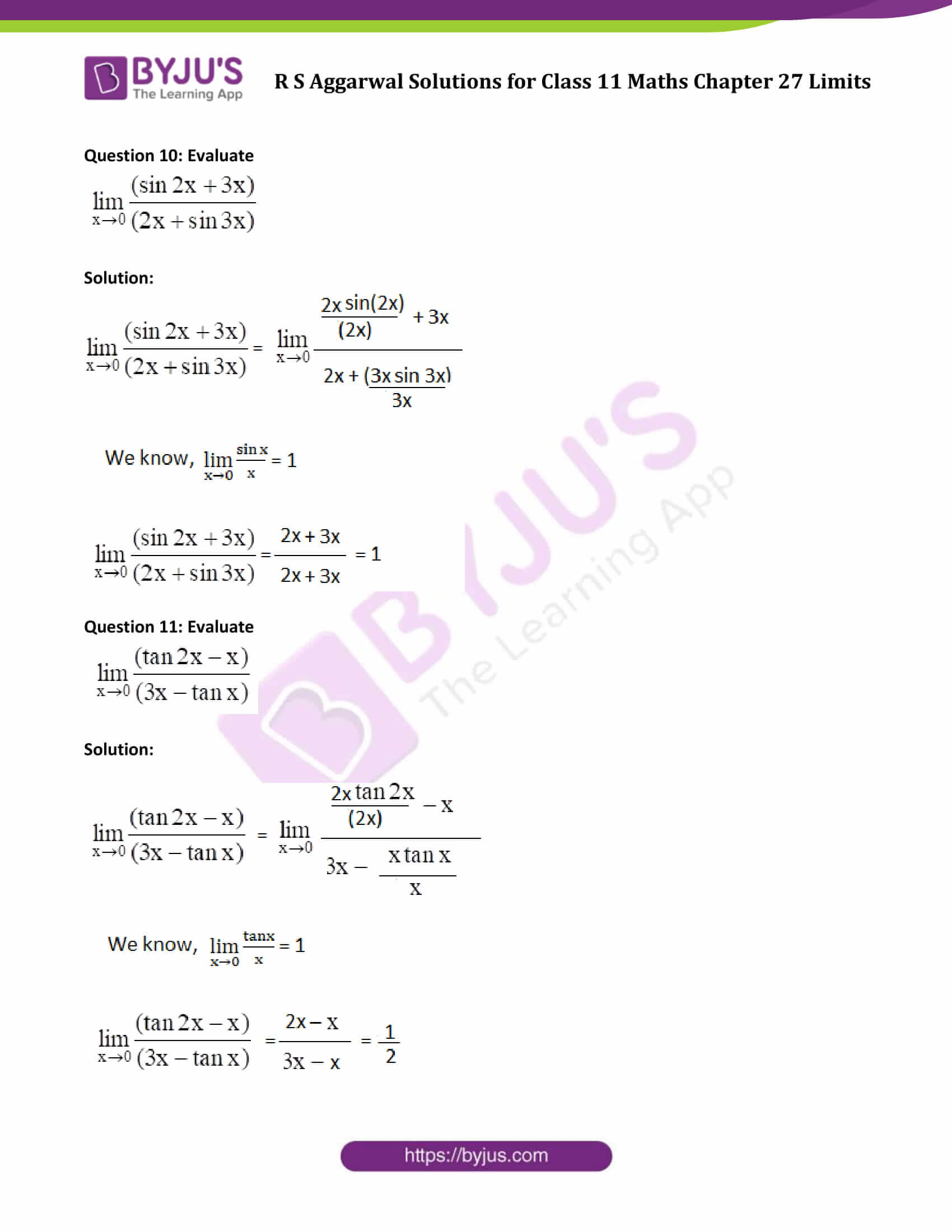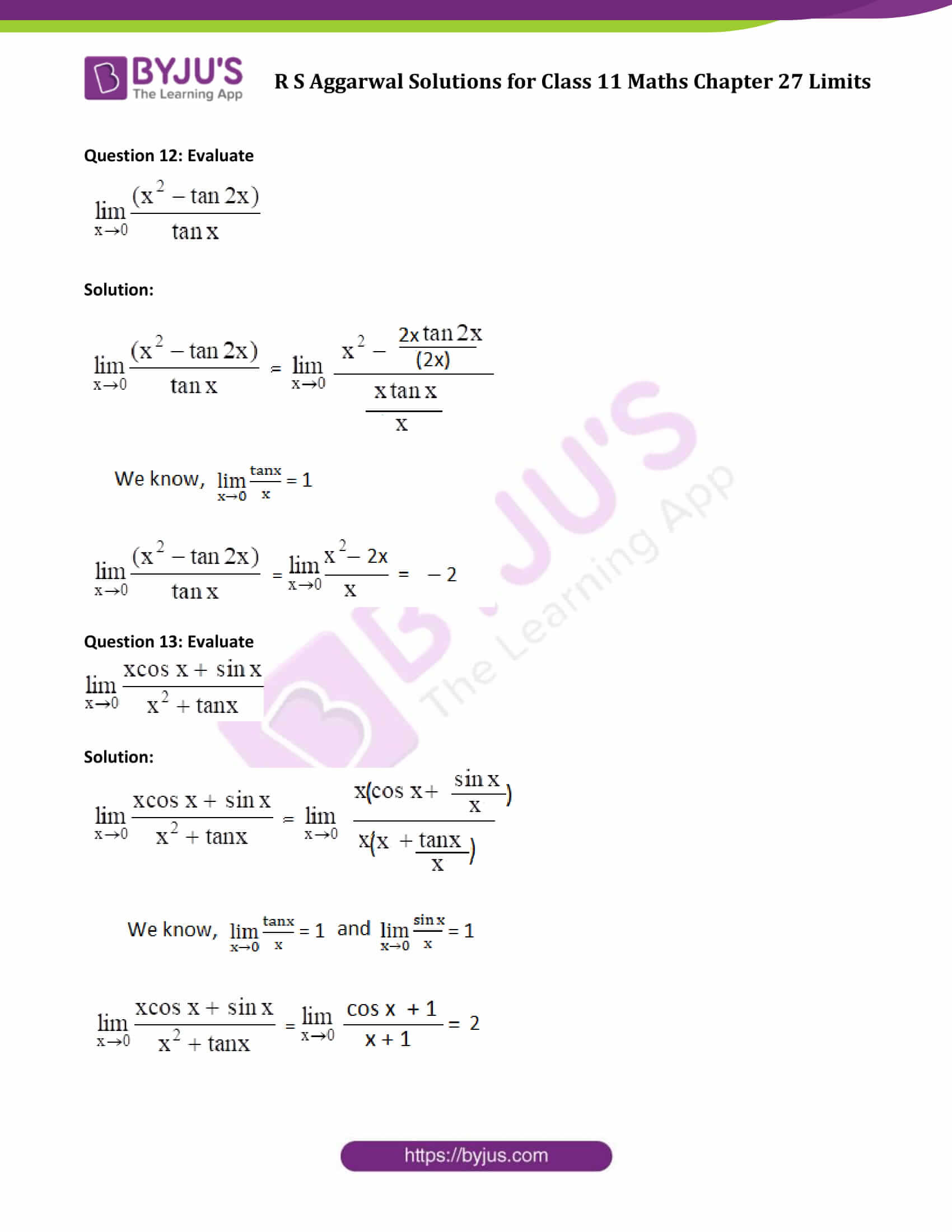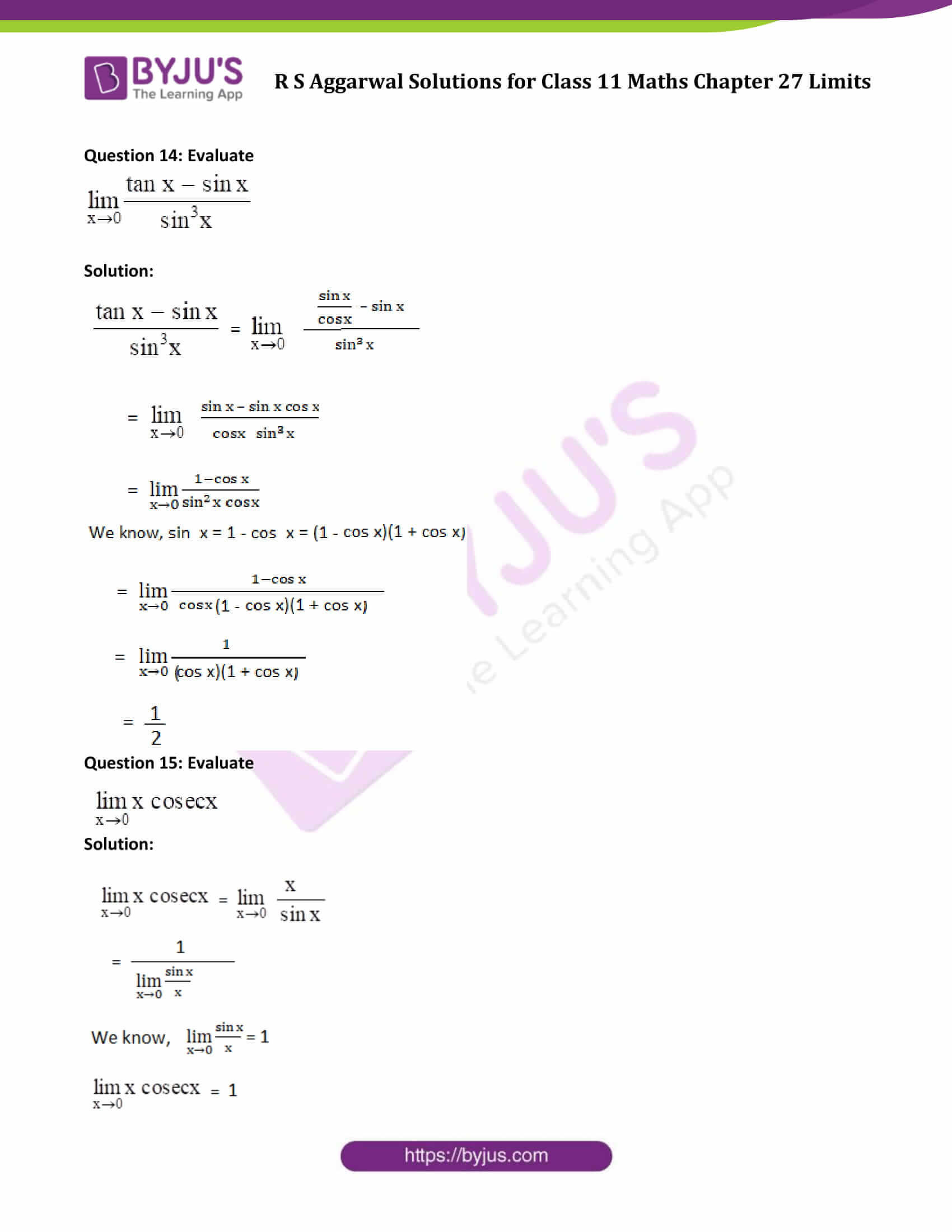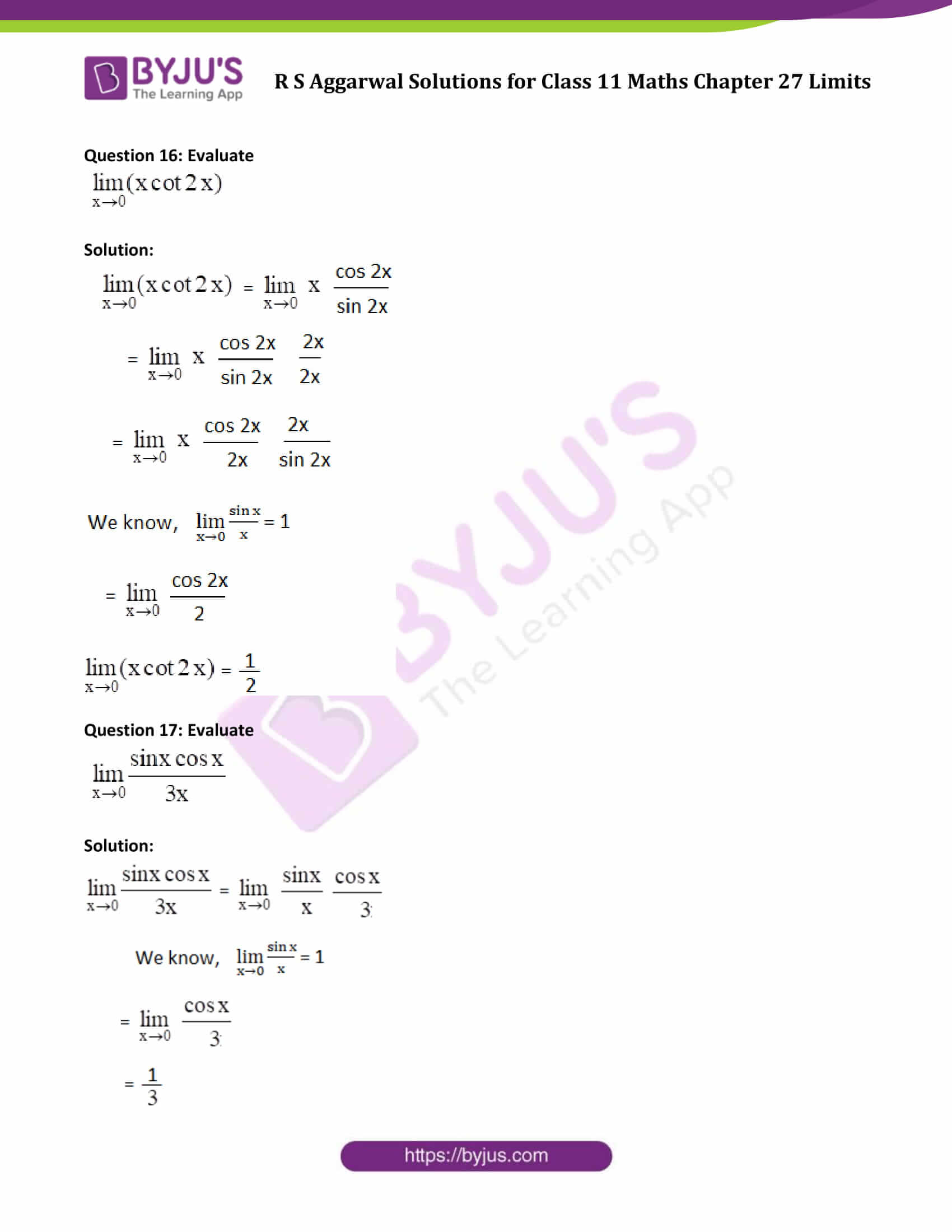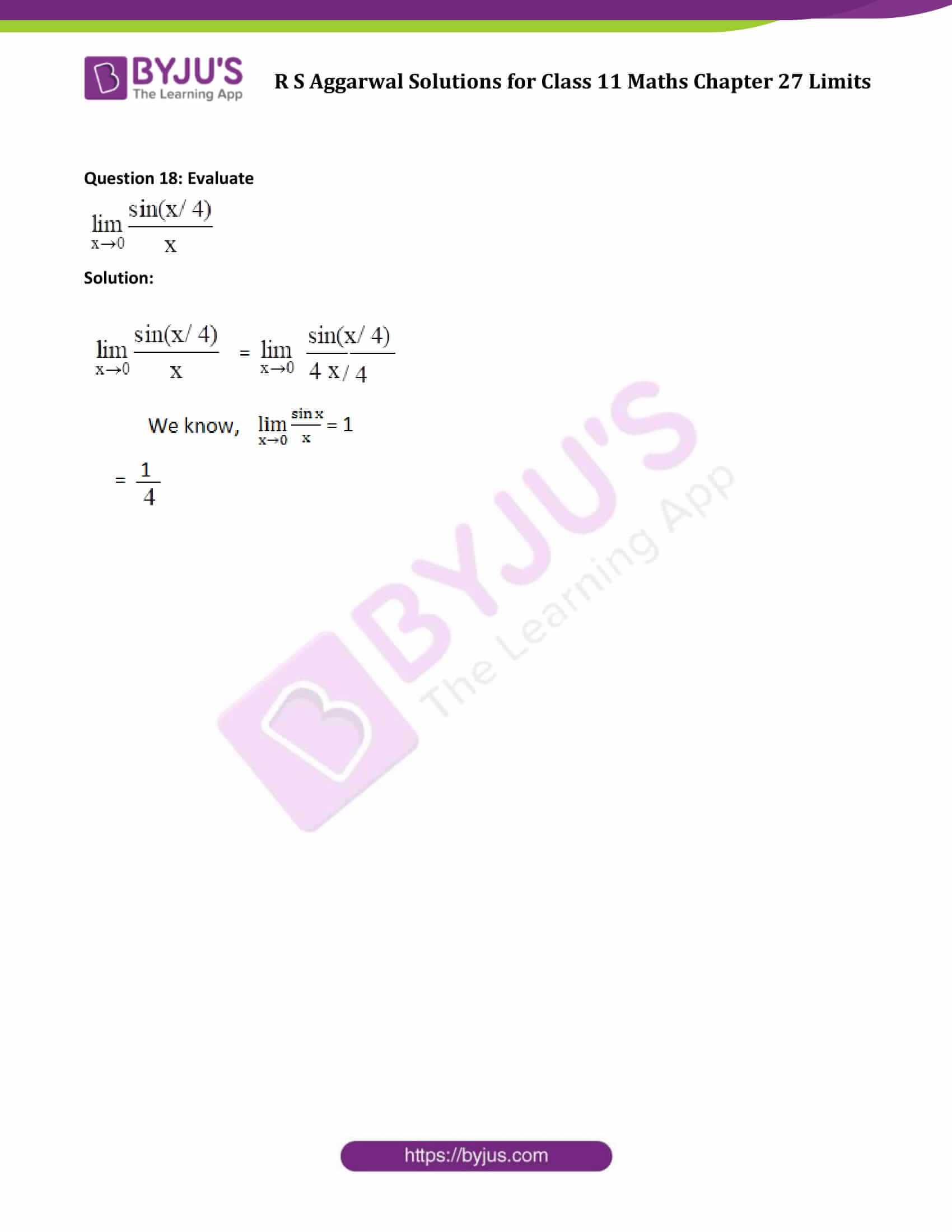### Access Answers of R S Aggarwal Solutions for Class 11 Maths Chapter 27 Limits Exercise 27B Page number 817

Question 1: Evaluate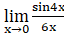Solution: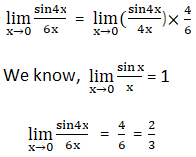Question 2: Evaluate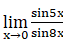Solution: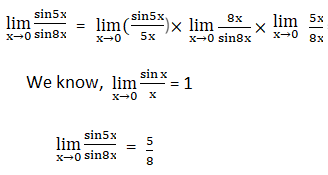Question 3: Evaluate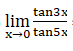Solution: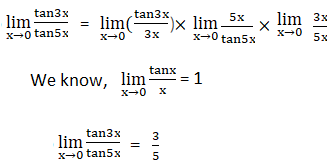Question 4: Evaluate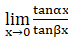Solution: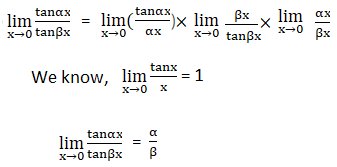Question 5: Evaluate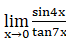Solution: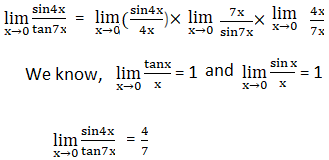Question 6: Evaluate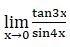Solution: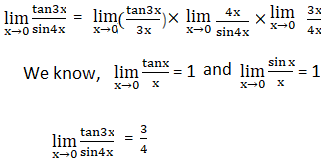Question 7: Evaluate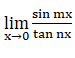Solution: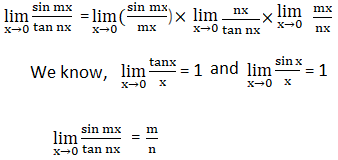Question 8: Evaluate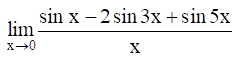Solution: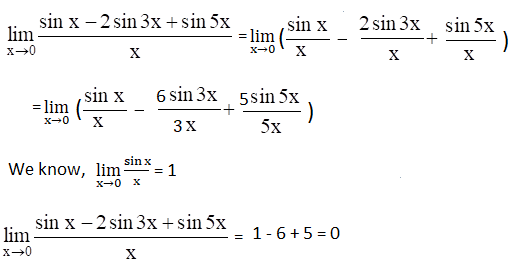Question 9: Evaluate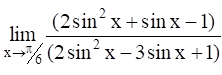Solution:

Let sin x = y

Numerator: 2y^2 + y – 1 = (2y – 1)(y + 1)

Denominator: 2y^2 – 3y + 1 = (2y – 1)(y – 1)

Eliminate common term from numerator and denominator which is (2y-1).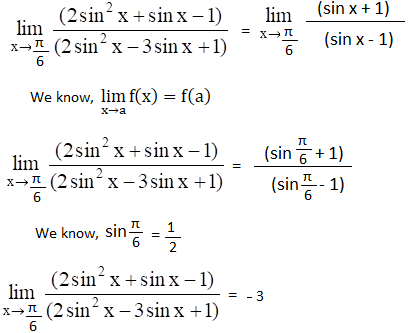Question 10: Evaluate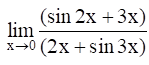Solution: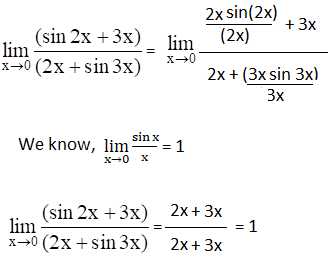Question 11: Evaluate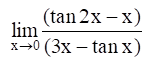Solution: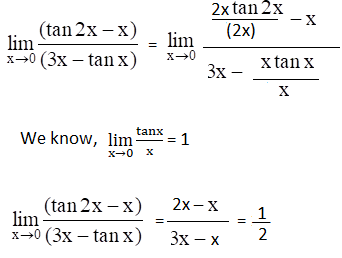Question 12: Evaluate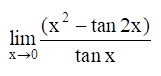Solution: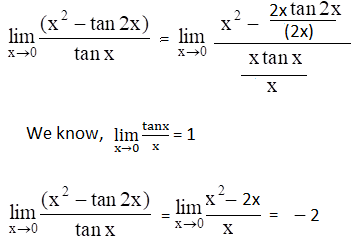Question 13: Evaluate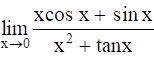Solution: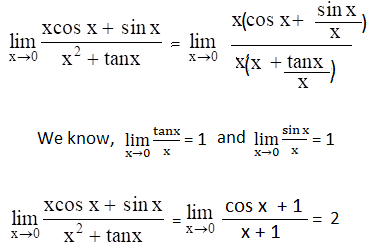Question 14: Evaluate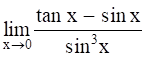Solution: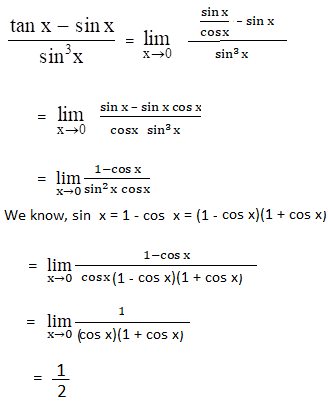Question 15: Evaluate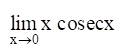Solution: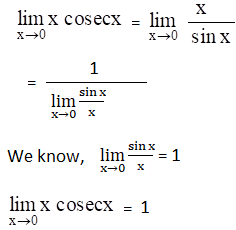Question 16: Evaluate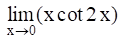Solution: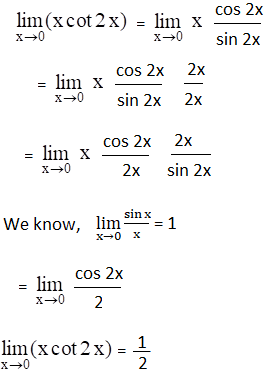Question 17: Evaluate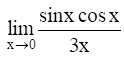Solution: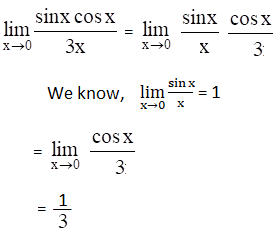Question 18: Evaluate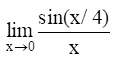Solution: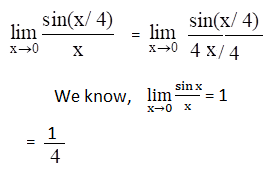## Access other exercise solutions of Class 11 Maths Chapter 27 Limits

Exercise 27A Solutions

Exercise 27C Solutions

## R S Aggarwal Solutions for Class 11 Maths Chapter 27 Exercise 27B

Class 11 Maths Chapter 27 Limits Exercise 27B is based on the topic – Trigonometric limits. Students can download R S Aggarwal class 11 solutions pdf and make use of the solutions to develop better Maths skills and prepare for exams.